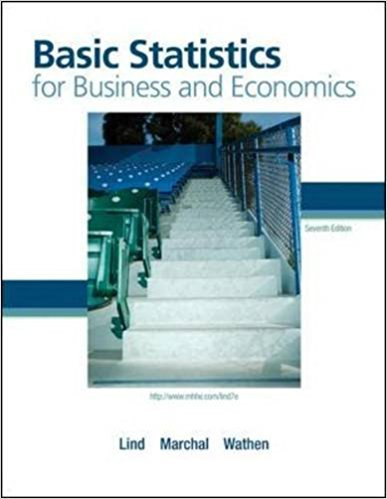×
×

# Listed below is the amount of commissions earned lastISBN: 9780077384470 64

## Solution for problem 28E Chapter 4

Basic Statistics for Business and Economics | 7th Edition

• Textbook Solutions
• 2901 Step-by-step solutions solved by professors and subject experts
• Get 24/7 help from StudySoup virtual teaching assistantsBasic Statistics for Business and Economics | 7th Edition

4 5 0 318 Reviews
15
5
Problem 28E

Listed below is the amount of commissions earned last month for the eight members of the sales staff at Best Electronics. Calculate the coefficient of skewness using both methods. Hint: Use of a spreadsheet will expedite the calculations.

 980.9 1,036.5 1,099.5 1,153.9 1,409.0 1,456.4 1,718.4 1,721.2
Step-by-Step Solution:
Step 1 of 3

Solution:

Step1 of 1:

The given data is

 980.9 1,036.50 1,099.50 1,153.90 1,409.00 1,456.40 1,718.40 1,721.20

Here we need to find the coefficient of skewness using both methods.

In this problem we don't have a mode. So we need to find another method.

That is,

The formula for pearson coefficient of skewness for the median isNow, we need to find mean, median and standard deviation.

Mean...

Step 2 of 3

Step 3 of 3

##### ISBN: 9780077384470

Unlock Textbook Solution